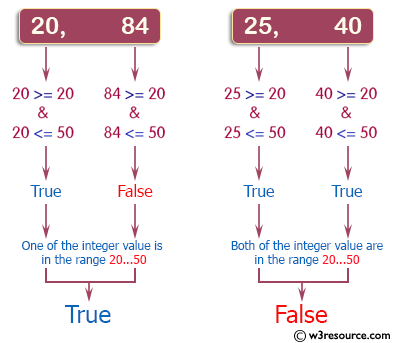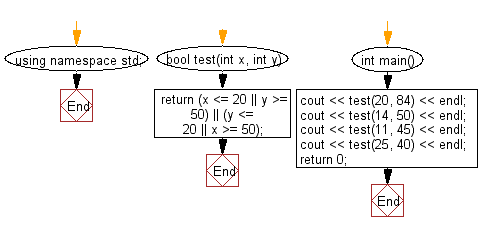﻿ C++ : Check if two values are in the range 20..50 inclusive# C++ Exercises: Check whether two given integer values are in the range 20..50 inclusive

## C++ Basic Algorithm: Exercise-16 with Solution

Write a C++ program to check whether two given integer values are in the range 20..50 inclusive. Return true if 1 or other is in the said range otherwise false.

Sample Solution:

C++ Code :

``````#include <iostream>

using namespace std;

bool test(int x, int y)
{
return (x <= 20 || y >= 50) || (y <= 20 || x >= 50);
}

int main()
{
cout << test(20, 84) << endl;
cout << test(14, 50) << endl;
cout << test(11, 45) << endl;
cout << test(25, 40) << endl;
return 0;
}
``````

Sample Output:

```1
1
1
0
```

Pictorial Presentation:Flowchart:C++ Code Editor:

Contribute your code and comments through Disqus.

What is the difficulty level of this exercise?

﻿